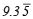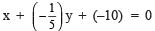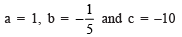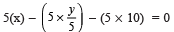# Ex 4.1 NCERT Solutions - Linear Equations in Two Variables Class 9 Notes | EduRev

## Class 9 : Ex 4.1 NCERT Solutions - Linear Equations in Two Variables Class 9 Notes | EduRev

The document Ex 4.1 NCERT Solutions - Linear Equations in Two Variables Class 9 Notes | EduRev is a part of the Class 9 Course Class 9 Mathematics by VP Classes.
All you need of Class 9 at this link: Class 9

Question 1. The cost of a notebook is twice the cost of a pen. Write a linear equation in two variables to represent this statement. (Take the cost of a notebook to be ₹ x and that of a pen to be ₹ y).
Solution:
Let the cost of a notebook = ₹ x
The cost of a pen = y
According to the condition,
we have [Cost of a notebook] = 2 x [Cost of a pen]
i.e. [x] = 2 x [y]
or
x = 2y
or
x – 2y = 0
Thus, the required linear equation is x – 2y = 0.

Question 2. Express the following linear equations in the form ax + by + c = 0 and indicate the values of a, b and c in each case:
(i) 2x + 3y =(ii) x – (y/5) – 10 = 0
(iii) –2x + 3y = 6
(iv) x = 3y
(v) 2x = –5y
(vi) 3x + 2 = 0
(vii) y – 2 = 0
(viii) 5 = 2x
Solution: (i) We have 2x + 3y =∴ (2)x + (3)y + (–) = 0
Comparing it with ax + bx + c = 0,
we have a = 2, b = 3 and c = –.

(ii) We have x –(y/5) – 10 = 0

orComparing with ax + bx + c = 0, we getNote: Above equation can also be compared by:

Multiplying throughout by 5,or 5x – y – 50 = 0
or 5(x) + (–1)y + (–50) = 0

Comparing with ax + by + c = 0,
we get a = 5, b = –1 and c = –50.

(iii) We have –2x + 3y = 6
⇒ –2x + 3y – 6 = 0
⇒ (–2)x + (3)y + (–6) = 0
Comparing with ax + bx + c = 0,
we get a = –2, b = 3 and c = –6.

(iv) We have x = 3y
⇒ x – 3y = 0
⇒ (1)x + (–3)y + 0 = 0
Comparing with ax + bx + c = 0,
we get a = 1, b = –3 and c = 0.

(v) We have 2x = –5y
⇒ 2x + 5y = 0
⇒ (2)x + (5)y + 0 = 0
Comparing with ax + by + c = 0,
we get a = 2, b = 5 and c = 0.

(vi) We have 3x + 2 = 0
⇒ 3x + 2 + 0y = 0
⇒ (3)x + (0)y + (2) = 0
Comparing with ax + by + c = 0,
we get a = 3, b = 0 and c = 2.

(vii) We have y – 2 = 0
⇒ (0)x + (1)y + (–2) = 0
Comparing with ax + by + c = 0,
we have a = 0, b = 1 and c = –2.

(viii) We have 5 = 2x
⇒ 5 – 2x = 0
⇒ –2x + 0y + 5 = 0
⇒ (–2)x + (0)y + (5) = 0
Comparing with ax + by + c = 0,
we get a = –2, b = 0 and c = 5.

Offer running on EduRev: Apply code STAYHOME200 to get INR 200 off on our premium plan EduRev Infinity!

132 docs

,

,

,

,

,

,

,

,

,

,

,

,

,

,

,

,

,

,

,

,

,

;